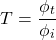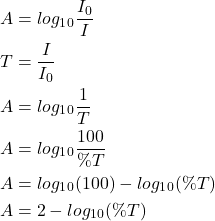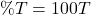# Transmittance (Formula & Transmittance to Absorbance Calculation)

Contents

## What is Transmittance?

The transmittance of a surface or material is defined as the part of the light that moves through the other side of the surface. When light passes through any surface or material, it can be transmitted, reflected, or absorbed. Transmittance and reflectance are closely related concepts.

Transmittance is defined as a ratio of the intensity of incident light (I0) to the amount of intensity passes through the object (I). The transmittance is denoted as T.As shown in the above figure, the I0 is the intensity of incident light. This light passes through the block of glass or any other material. The I is the intensity of light that can pass through the material.

The transmittance is a ratio of intensity. Therefore, the transmittance has no unit.

Let’s understand the transmittance by an example.

If you pass the light from a semi-transparent block of glass. Let say 30% of light is reflected from the surface of the glass. Remaining 70% of the light will try to pass the block of glass. But, some amount of light will be absorbed by the molecules of light. Let say it is 35% of light. Now the remaining 35% light will pass from the other side of the block. It means that the glass block has a transmittance of 35%.

## Transmittance Formula

Transmittance is also defined in terms of radiant flux. The ratio of radiant flux transmitted by surface (фt) to the radiant flux received by that surface (фi) is known as the Transmittance (T). So, the formula of transmittance is;## Transmittance to Absorbance

The amount of radiation absorbed can be measured by the transmittance. The relation between transmittance (T) and absorbance (A) is given by the Beer-Lambert law (Beer’s law).

According to Beer’s Law, the absorbance is formulated as:Let’s assume a condition in which the light passes through the object without any absorbance, it means 100% light will pass through the object. So, in this condition, the transmittance is 100%.

From the equation of Beer’s law, we can calculate the absorbance and it is zero.

Now assume the opposite condition – the light cannot pass the object. In this condition, the transmittance is zero and the absorbance is infinite.

## Absorbance vs Transmittance

Absorbance and transmittance both terms are opposite to each other. The difference between these two terms is summarized in the table below.

## What is Percentage Transmittance

Transmittance measures the amount of light that can pass through the material. Percentage transmittance is defined as the percentage of light that can be transmitted from the other side of the surface.

The equation of percentage transmittance (%T) is as### What Does a High Percent Transmittance Mean

The value of percentage transmittance provides information about surface or material used in various applications.

If the value of percentage transmittance is high, it means that the surface will allow more lights to pass. Similarly, if the value of percentage transmittance is low, it means that the surface absorbs the more amount of light.

For a perfectly transparent material, the 100% light will pass through the other side of martial and the transmittance is 100% for this type of material.

For a completely opaque material, the light cannot pass through the material. Therefore, the transmittance is 0%.

So, high the value of percentage transmittance gives higher the transparency of the material.

## Why is Absorbance the Preferred Unit Over Transmittance?

The absorbance and transmittance are related to each other. The old IR-spectra can only be found in Transmittance mode. So, it only measures the Transmittance. But, after the use of microprocessors, it automatically converts transmittance into absorbance.

The use of absorbance and transmittance is a personal preference.

Absorbance is a logarithmic scale of Transmittance. The absorbance is enabled to detect weak signals next to pass signals.

And also, the absorbance is proportional to analytic concentration whereas the transmittance is not proportional to analytic concentration.

## What is the Use of Transmittance (Applications)

The measurement of transmittance has many applications, including:

• Measuring concentrations of chemicals in solutions
• Water clarity